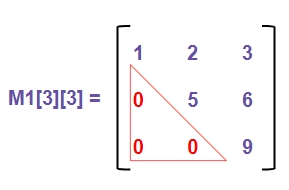# Program to check if matrix is upper triangular in C++

Given a square matrix M[r][c] where ‘r’ is some number of rows and ‘c’ are columns such that r = c, we have to check that ‘M’ is upper triangular matrix or not.

## Upper Triangular Matrix

Upper triangular matrix is a matrix in which the elements above the main diagonal(including the main diagonal) are not zero and below elements are zero only.

Like in the given Example below −In above figure the red highlighted elements are lower elements from the main diagonal which are zero and rest elements are non-zero.

## Example

Input: m = { {1, 2, 3},
{0, 5, 6},
{0, 0, 9}}
Output: yes
Input: m == { {3, 0, 1},
{6, 2, 0},
{7, 5, 3} }
Output: no

## Algorithm

Start
Step 1 -> define macro as #define size 4
Step 2 -> Declare function to check matrix is lower triangular matrix
bool check(int arr[size][size])
Loop For int i = 1 and i < size and i++
Loop For int j = 0 and j < i and j++
IF (arr[i][j] != 0)
return false
End
End
End
Return true
Step 3 -> In main()
Declare int arr[size][size] = { { 1, 1, 3, 2 },
{ 0, 3, 3, 2 },
{ 0, 0, 2, 1 },
{ 0, 0, 0, 1 } }
IF (check(arr))
Print its a lower triangular matrix
End
Else
Print its not a lower triangular matrix
End
Stop

## Example

#include <bits/stdc++.h>
#define size 4
using namespace std;
// check matrix is lower triangular matrix
bool check(int arr[size][size]){
for (int i = 1; i < size; i++)
for (int j = 0; j < i; j++)
if (arr[i][j] != 0)
return false;
return true;
}
int main(){
int arr[size][size] = { { 1, 1, 3, 2 },
{ 0, 3, 3, 2 },
{ 0, 0, 2, 1 },
{ 0, 0, 0, 1 } };
if (check(arr))
cout << "its a lower triangular matrix";
else
cout << "its not a lower triangular matrix";
return 0;
}

## Output

its a lower triangular matrix